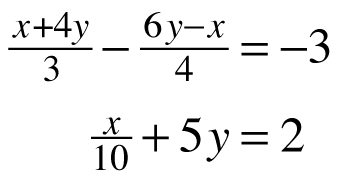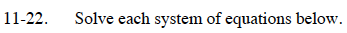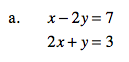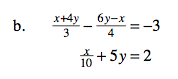Home > CCA2 > Chapter 11 > Lesson 11.1.2 > Problem11-22

11-22.
1. Solve each system of equations below. Homework Help ✎

1. x − 2y = 7
2x + y = 3

2.$\left(\frac{13}{15}, -\frac{11}{5}\right)$4x + 16y − 18y + 3x = −36
x + 50y = 20

7x − 2y = −36
x = 20 − 50y

7(20 − 50y) − 2y = −36

$y =\frac{1}{2}$

$\frac{x}{10}+5\left(\frac{1}{2}\right)=2$

x = −5

$\left(-5, \frac{1}{2}\right)$